• 题意：按顺时针或逆时针给出多边形的n个顶点，问该多边形是否是一个轴对称图形； 思路：对于奇顶点多边形，其对称轴一定经过某一边的中点和其对边的连线；  对于偶顶点多边形，其对称轴要么经过两相对顶点的连线...
Swordsman
题目链接：HDU - 3902
题意：按顺时针或逆时针给出多边形的n个顶点，问该多边形是否是一个轴对称图形；
思路：对于奇顶点多边形，其对称轴一定经过某一边的中点和其对边的连线；
对于偶顶点多边形，其对称轴要么经过两相对顶点的连线，要么经过两相对边的中点的连线；
但是总体来说，若某一多边形是轴对称图形，那么在该多边形上的任意相对的两点连线被对称轴垂直平分；
所以加入边的中点，枚举任何一个可能是对称轴的两点两线，判断；
#include <bits/stdc++.h>
#define esp 1e-10
using namespace std;
const int maxn=20100;
struct point{
double x, y;
}p[maxn<<1], a[maxn];
double cross(point a, point b, point c){
return (b.x-a.x)*(c.y-a.y)-(b.y-a.y)*(c.x-a.x);
}
double pcross(point a, point b, point c, point d){
return (a.x-b.x)*(c.x-d.x)+(a.y-b.y)*(c.y-d.y);
}
double mid(double a, double b){
return (a+b)/2.0;
}
bool check(point a, point b, point c, point d){
point e=(point){mid(c.x, d.x), mid(c.y, d.y)};
return fabs(pcross(c, d, a, b))<esp && fabs(cross(a, b, e))<esp;
}
int main(){
int n;
while(~scanf("%d", &n)){
for(int i=0; i<n; i++){
scanf("%lf%lf", &a[i].x, &a[i].y);
}
a[n]=a;
int cnt=0;
for(int i=1; i<=n; i++){
p[cnt++]=a[i-1];
p[cnt++]=(point){mid(a[i-1].x, a[i].x), mid(a[i-1].y, a[i].y)};
}
int t=cnt/2;
int flag=0;
for(int i=0; i+t<cnt; i++){
int j, k;
for(j=i+t-1, k=1; i<j; j--, k++){
if(!check(p[i], p[i+t], p[j], p[(i+t+k)%cnt])) break;
}
if(i==j){
flag=1;
break;
}
}
if(flag) printf("YES\n");
else printf("NO\n");
}
return 0;
}


展开全文• %% 直线为中心对称的圆clc;clear;hold onsyms x y;% 画对称轴x1=-20:0.1:20;k=1;b1=10;f1(x)=k.*x+b1;plot(x1,f1(x1));theta=0:2*pi/3600:2*pi;R=4;Circle1=10+R*cos(theta);Circle2=10+R*sin(theta);plot(Circle1...

%% 直线为轴中心对称的圆
clc;
clear;
hold on
syms x y;
% 画对称轴
x1=-20:0.1:20;
k=1;
b1=10;
f1(x)=k.*x+b1;
plot(x1,f1(x1));
theta=0:2*pi/3600:2*pi;
R=4;
Circle1=10+R*cos(theta);
Circle2=10+R*sin(theta);
plot(Circle1,Circle2,'m','Linewidth',3);
x1=Circle1;
y1=Circle2;
% 对称点坐标(2*x0-x1,2f(x0)-y1)
b0=y1+(1/k).*x1;
x0=(b0-b1).*(k/(k^2+1));
x2=2*x0-x1;
y2=2*f1(x0)-y1;
plot(x2,y2,'r-');
axis equal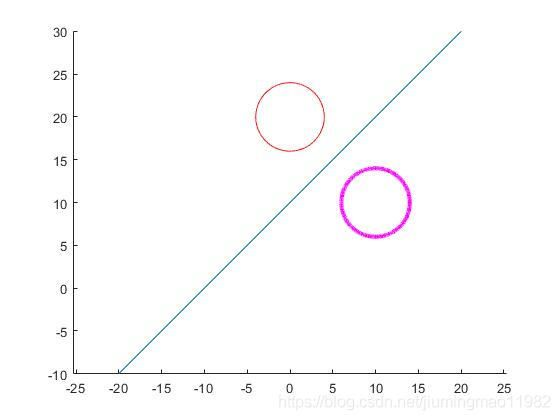%% 直线为轴中心对称的三角形
close all; clear all; clc
hold on
x1=-20:0.1:20;
syms x
k=1/2;
b1=4;
f1(x)=k.*(x+2)+b1;
plot(x1,f1(x1),'b');
x1=1;x2=2;x3=1;
y1=1;y2=2;y3=3;
triangle_x1=[x1,x2,x3,x1];
triangle_y1=[y1,y2,y3,y1];
fill(triangle_x1,triangle_y1,'r');
% 对称点坐标(2*x0-x1,2f(x0)-y1)
b0=triangle_y1+(1/k).* triangle_x1;
x0=(b0-b1).*(k/(k^2+1));
triangle_x2=2*x0-triangle_x1;
triangle_y2=2*f1(x0)-triangle_y1;
% triangle_y2=[1,2,1,1];
fill(triangle_x2,triangle_y2,'b');
axis([-5 5 -5 30])
axis equal;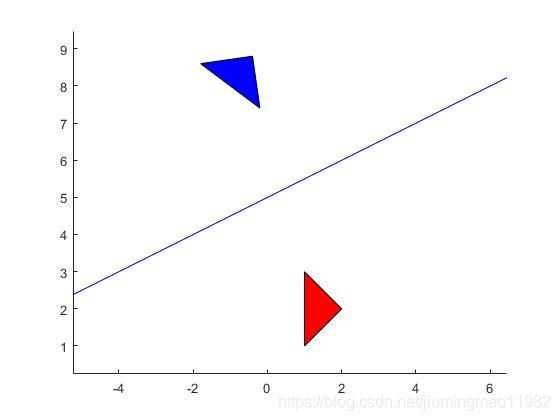%% 点为中心对称的三角形
close all; clear all; clc
hold on
x0=1.5;
y0=2;
plot(x0,y0,'*')
x1=1;x2=2;x3=1;
y1=1;y2=2;y3=3;
triangle_x1=[x1,x2,x3,x1];
triangle_y1=[y1,y2,y3,y1];
fill(triangle_x1,triangle_y1,'r');
triangle_x2=2*x0-triangle_x1;
triangle_y2=2*y0-triangle_y1;
fill(triangle_x2,triangle_y2,'b');
axis equal;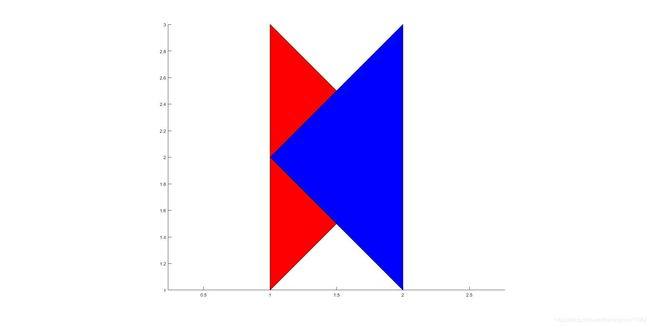展开全文• 开发环境:Turbo C 2.0 * ******************** *** *题目很简单就是输出如图所示的图形图形用你喜欢的图形输出即可:*，~，-，+等。当初做这样的题目的时候我们是在学循环，因此是要我们用循环的思想来解决问题...
开发环境:Turbo C 2.0   *  ********************  ***   *题目很简单就是输出如图所示的图形，图形用你喜欢的图形输出即可:*，~，-，+等。当初做这样的题目的时候我们是在学循环，因此是要我们用循环的思想来解决问题，而不是直接把图形输出。我们先把这个图形用*表示出来   *  ********************  ***   *我先给每行标上号(请注意下怎么标号的)   *       -3 //有一个图    ***      -2 //有三个图  *****     -1 //有五个图  *******    0  //有七个图  *****     1  //有五个图    ***      2  //有三个图     *       3 //有一个图  这里值得注意的就是:中间图最多为七，也就是最大行数的数字(规律一:得到数字7[最大行数])标的号的绝对值就是每行前面空的格数。由于图形的特殊型，它必须是基数行的，所以标的号始终是 [-(7-1)/2,(7-1)/2]之间(规律二：循环是从-(最大行数-1)/2到(最大行数-1)/2)由中间向两边展开始终是少两个，又根据标号的规律性可知由中间向两边的输出图形数为:(最大行数-2*|标号|)标号也就是循环数总数三大规律我们可知道，如果把输出图形写作一个函数的话，那么它只需传入一个参数，那就是最大行数。void print(int maxnumber){  int i = 0, j = 0;  for (i = -((maxnumber-1)/2); i <= ((maxnumber-1)/2); i ++)  {    for (j = 0; j < abs(i); j ++)//输出空格    {      printf(” “);    }        for ( j = 0; j < (maxnumber-2*abs(i)); j ++)    {      printf(”*”);    }    printf(”/n”);  }}我的完整程序如下:#include<stdio.h>
void print(int maxnumber);
int main(void){  printline(9);  return 0;}
void print(int maxnumber){  int i = 0, j = 0;  for (i = -((maxnumber-1)/2); i <= ((maxnumber-1)/2); i ++)  {    for (j = 0; j < abs(i); j ++)    {      printf(” “);    }    for (j = 0; j < (maxnumber-2*abs(i)); j ++)    {      printf(”*”);    }    printf(”/n”);  }}
个人永久博客:Chenliang’s blog
C语言对于轴对称图形输出的分析

展开全文语言 c
• %% 直线为中心对称的圆 clc; clear; hold on syms x y; % 画对称轴 x1=-20:0.1:20; k=1; b1=10; f1(x)=k.*x+b1; plot(x1,f1(x1)); theta=0:2*pi/3600:2*pi; R=4; Circle1=10+R*cos(theta); Circle2=10+R*sin(theta...
%% 直线为轴中心对称的圆 clc; clear; hold on syms x y; % 画对称轴 x1=-20:0.1:20; k=1; b1=10; f1(x)=k.*x+b1; plot(x1,f1(x1)); theta=0:2*pi/3600:2*pi; R=4; Circle1=10+R*cos(theta); Circle2=10+R*sin(theta); plot(Circle1,Circle2,'m','Linewidth',3); x1=Circle1; y1=Circle2; % 对称点坐标(2*x0-x1,2f(x0)-y1) b0=y1+(1/k).*x1; x0=(b0-b1).*(k/(k^2+1)); x2=2*x0-x1; y2=2*f1(x0)-y1; plot(x2,y2,'r-'); axis equal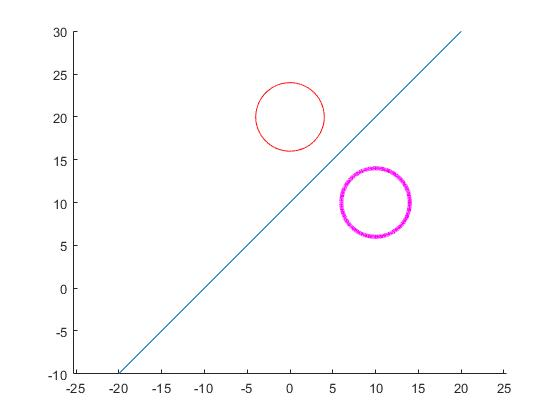%% 直线为轴中心对称的三角形 close all; clear all; clc hold on x1=-20:0.1:20; syms x k=1/2; b1=4; f1(x)=k.*(x+2)+b1; plot(x1,f1(x1),'b');
x1=1;x2=2;x3=1; y1=1;y2=2;y3=3; triangle_x1=[x1,x2,x3,x1]; triangle_y1=[y1,y2,y3,y1]; fill(triangle_x1,triangle_y1,'r');
% 对称点坐标(2*x0-x1,2f(x0)-y1) b0=triangle_y1+(1/k).* triangle_x1; x0=(b0-b1).*(k/(k^2+1)); triangle_x2=2*x0-triangle_x1; triangle_y2=2*f1(x0)-triangle_y1; % triangle_y2=[1,2,1,1]; fill(triangle_x2,triangle_y2,'b'); axis([-5 5 -5 30]) axis equal;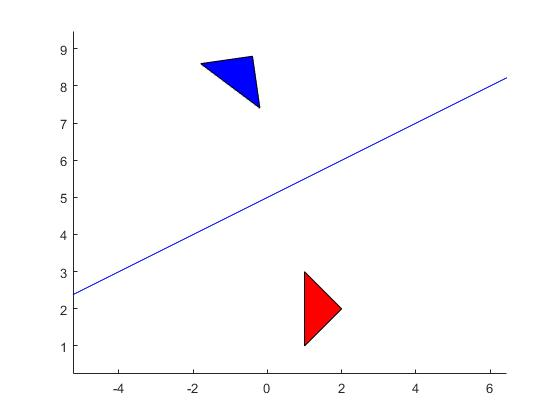%% 点为中心对称的三角形 close all; clear all; clc hold on x0=1.5; y0=2; plot(x0,y0,'*') x1=1;x2=2;x3=1; y1=1;y2=2;y3=3; triangle_x1=[x1,x2,x3,x1]; triangle_y1=[y1,y2,y3,y1]; fill(triangle_x1,triangle_y1,'r'); triangle_x2=2*x0-triangle_x1; triangle_y2=2*y0-triangle_y1; fill(triangle_x2,triangle_y2,'b'); axis equal;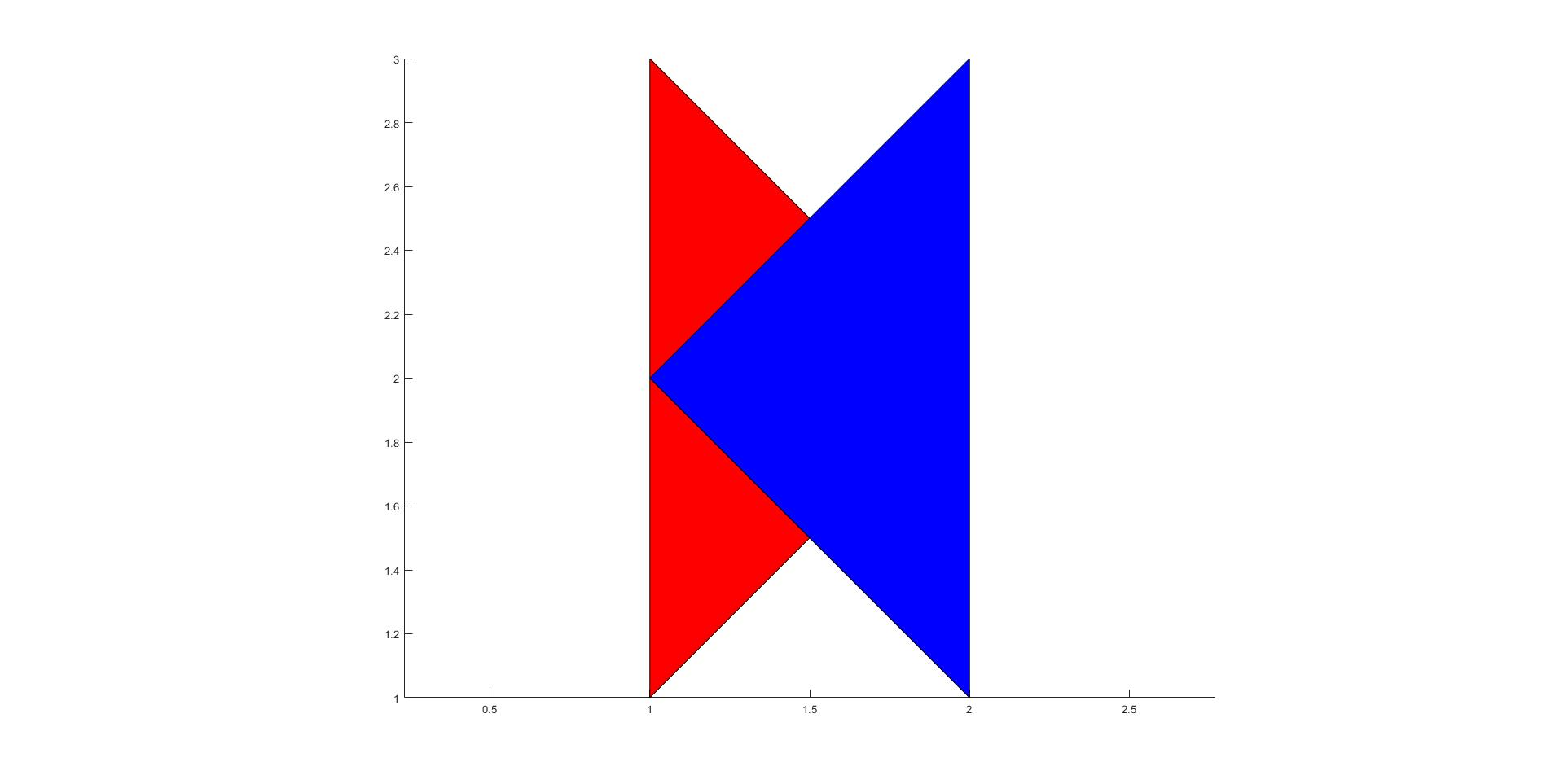展开全文matlab 矩阵 算法
• 之前做这类题目的时候是先找多边形的重心，因为对称轴一定经过重心，然后再找另外一个点确定对称轴后判断 这次直接给每条线段添加一个中点，然后开始枚举最多n条对称轴，然后判断是否对称 也就是对称轴一定垂直...HDU
• 这题看到的时候顿时蛋疼了，我在FJNU的比赛和长沙的华南赛都遇到过类似的题目，FJNU的比赛各边是和x,y轴都是平行的，只需枚举4个方向的对称轴，长沙和这题很相似，要枚举所有对称轴，数据比较小，这题数据比较大，...c
• 核心方法： public Bitmap rotate(Bitmap b, int degrees){ if(degrees != 0 && b != null){ Matrix m = new Matrix();... m.setRotate(degrees, b.getWidth(), b....这样t2 就得到了t1的对称Y轴图片了。android matrix exception float
• # 背景#### 材料力学...----------# 说明#### 由于还没有开发完全，本程序支持的横截面至少有一个与坐标垂直的对称轴----------# 测试## 测试1#### ![](https://leanote.com/api/file/getImage?fileId=58fb0a15...
• 判断n边形是否是轴对称图形： n边形的n个顶点再加上n条边的中点，共2*n个顶点。 如果存在对称轴,必定是点i和点i+n连成的直线，然后分别验证两边对称的点 到点i和点i+n的距离是否相等 */ #include < stdio.h > #...
• 关于图形y轴旋转的体积的记录 图形可拆为一层包含一层的圆圈（类似于不同型号的水管嵌套），至于公式咋来的可以这样推导： 每一层的体积dV = 底面积 * 高 (因为是圆柱掏空，所以公式和圆柱体是一样的) 在∆x→0...
• ERRORBARLOG(X,Y,E,...) 使用对数 Y 绘制向量 X 与向量 Y图形，使用关于数据点的对称条，即：条使得 Y 是几何平均值（而不是算术平均值）下条和上条。 误差棒的总长度为 2E。 ERRORBARLOG 与原始 Matlab 的 ...matlab
• app.title = '折柱混合'; option = { tooltip: { trigger: 'axis', axisPointer: { type: 'cross', crossStyle: { color: '#999' } ...
• 简介：可以自建模块，并调用模块内的函数绘制图形，判断这些图形是否为轴对称图形，并为轴堆成图形绘制出对称轴。 import turtle ------------------------------------------任务一------------------------------...map import文件
• 题意：给出一个图形的n个点，判断是否是轴对称图形 题解：对称轴平分图形，要么垂直平分某条边(或者两条边)，其他点关于对称轴对称，要么对称轴经过某个点(或者两个),其他点关于对称轴对称，枚举边和点，点积判断下...
• 在Python的数据可视化库中，采用matplotlib绘制相关图形时，若不加任何设定，一般的x-y坐标是不带箭头且是一个封闭的矩形。我们以Sigmoid函数的绘制，给大家展示一下。import matplotlib.pyplot as plt #导入...
• 前段时间有个图形学的课程作业，花了点时间做了出来，今天在这里分享一下供后来者学习、借鉴（其实就是粘贴复制，大家懂的，嘿嘿）。 首先，声明一下所用的环境为：VS2015+EasyX图形库。因为要实现绘图，最好有专门...C++
• 分析出椭圆右上部分、右下部分的中点画线方法，并将所得点关于y轴对称，得出整个椭圆图案。 二、代码实现 待补 /* 程序：中点画线法实现焦点在x\y轴上的椭圆绘制 编译环境：Visual C++ 6.0 EasyX_20200109(beta) ...
• 轴对称问题 (当用三维模型仿真计算时间太长时，将它转化为二维模型，x轴为径向方向，y轴为旋转轴) 轴对称模型几何建立有两大要素，分别为 1.平面必须建立在全局坐标平面X-Y的第一、二象限内； 2.全局坐标Y轴为原始...ansys 经验分享
• 在Python的数据可视化库中，采用matplotlib绘制相关图形时，若不加任何设定，一般的x-y坐标是不带箭头且是一个封闭的矩形。我们以Sigmoid函数的绘制，给大家展示一下。import matplotlib.pyplot as plt #导入...python画箭头
• /* hdu 3902 Swordsman 题解：有点小暴力，但也很快A了。...再判断每一对点是否关于对称（可以根据其到i点的距离是否相等&&到i+n点距离是否相等判断）； */ #include #include using namespaiostream struct
• 接着，点排列下面的水平居中，这个道理其实就是将轴线当参考系，其他的图形以他为标准，进行水平居中。 如下图，将下面的矩形和上面的矩形对齐。 至于，左对齐，右对齐，都是可以的。 ...visio
• 2.关于任意平行于y轴直线图像的对称 3.关于任意点的对称 4.关于直线y=x对称 5.关于直线y=-x对称 编译器：vs2013 1 // x对称.cpp : 定义控制台应用程序的入口点。 2 // 3 4 #include "stdafx.h" 5...
• 1 #include<stdio.h> 2 3 main() 4 { 5 int i,p,n,k,f,c,h,g,w; 6 int a; 7 8 for(i=0;i<=8;i++) 9 { 10 for(p=0;p<=8;p++) 11 { 12 a[i][p...
• 在绘图时，我们有在一张图上绘制两条曲线的需求，但是两条曲线的y值相差较大，此时，可以选择绘制双y轴解决该问题。 二、yyaxis功能 创建具有两个 y 轴的图 三、函数 1、yyaxis left 激活当前坐标区中与左侧y轴...matlab
• 2.全局坐标Y轴为原始模型的旋转轴，全局坐标X轴为原始模型的径向方向。 选择create中的body transformation中的旋转rotate，就可将模型中的任何实体进行旋转，选择所剩的两个面，然后选择一个坐标轴，在angle...
• if(f[i].y==f[j].y||(f[i].x==f[j].x))//y坐标相等或者横坐标相等（因为当横坐标不相等，y坐标相等时，这两点事对称的，这是一种情况，还有就是当横坐标相等时，他也是对称的，因为是平行于y轴啊），下面偶数情况也...
• Matplotlib简介Matplotlib是非常强大的python画图工具...Matplotlib安装pip3 install matplotlib#python3双X轴的可以理解为共享y轴ax1=ax.twiny()ax1=plt.twiny()双Y轴的可以理解为共享x轴ax1=ax.twinx()ax1=p...
• 以绘制最简单的sin x曲线为例，输入以下代码： import numpy as np ...现希望x轴和y轴在零点相交，可以通过axis函数设置： import numpy as np import matplotlib.pyplot as plt x = np.arange(0, 6, 0python matplotlib
• echarts柱状图,设置上下Y轴,X轴在中间,柱子实现向上以及向下延伸,...